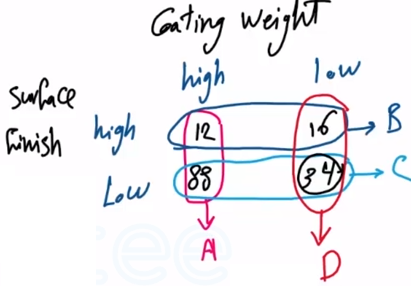Need Help?

Subscribe to Probability

###### \${selected_topic_name}
• Notes

The following table summarizes the analysis of samples
of galvanized steel for coating weight and surface roughness:

$\begin{array}{llll}{} & {} & {\frac{\text { coating weight }}{\text { high }}} & {\text { low }} \\ {\text { surface }} & {\text { high }} & {\text { 12 }} & {\text { 16 }} \\ {\text { roughness }} & {\text { low }} & {88} & {\text { 34 }}\end{array}$

(a) If the coating weight of a sample is high, what is the prob-
ability that the surface roughness is high?
(b) If the surface roughness of a sample is high, what is the
probability that the coating weight is high?
(c) If the surface roughness of a sample is low, what is the
probability that the coating weight is low?Coating weight high $\rightarrow A$

Surface finish high $\rightarrow B$

(a) $P(B | A)$

$P(B | A)=\frac{P(A \cap B)}{P(A)}$

$=\frac{n(A \cap B)}{n(A)}=\frac{12}{12+88}=0.12$

(b) $P(A | B) \Rightarrow P(A | B)=\frac{n(A \cap B)}{n(B)}$

$=\frac{12}{12+16} = \frac{12}{28}$

(c) surface roughness low $\rightarrow C$

coating weight low $\rightarrow d$

$P(D | C)=\frac{P\left(D \cap C\right)}{P(C)}$

$=\frac{34}{34+88}=\frac{34}{122} \simeq 0.28$